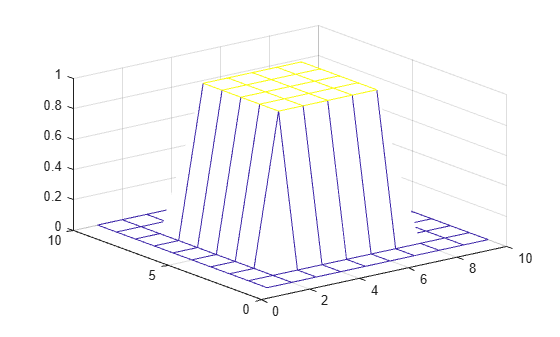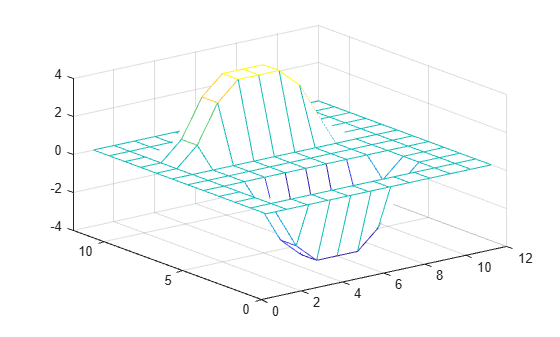Documentation

### This is machine translation

Mouseover text to see original. Click the button below to return to the English version of the page.

Note: This page has been translated by MathWorks. Click here to see
To view all translated materials including this page, select Country from the country navigator on the bottom of this page.

# filter2

2-D digital filter

## Syntax

``Y = filter2(H,X)``
``Y = filter2(H,X,shape)``

## Description

example

````Y = filter2(H,X)` applies a finite impulse response filter to a matrix of data `X` according to coefficients in a matrix `H`.```

example

````Y = filter2(H,X,shape)` returns a subsection of the filtered data according to `shape`. For example, `Y = filter2(H,X,'valid')` returns only filtered data computed without zero-padded edges.```

## Examples

collapse all

You can digitally filter images and other 2-D data using the `filter2` function, which is closely related to the `conv2` function.

Create and plot a 2-D pedestal with interior height equal to one.

```A = zeros(10); A(3:7,3:7) = ones(5); mesh(A)```Filter the data in `A` according to a filter coefficient matrix `H`, and return the full matrix of filtered data.

```H = [1 2 1; 0 0 0; -1 -2 -1]; Y = filter2(H,A,'full'); mesh(Y)```Rotate `H` 180 degrees and convolve the result with `A`. The output is equivalent to filtering the data in `A` with the coefficients in `H`.

```C = conv2(A,rot90(H,2)); mesh(C)```## Input Arguments

collapse all

Coefficients of the rational transfer function, specified as a matrix.

Data Types: `double` | `single` | `int8` | `int16` | `int32` | `int64` | `uint8` | `uint16` | `uint32` | `uint64` | `logical`
Complex Number Support: Yes

Input data, specified as a matrix. If one or both of `X` and `H` are of type `single`, then the output is also of type `single`. Otherwise, `filter2` returns type `double`.

Data Types: `double` | `single` | `int8` | `int16` | `int32` | `int64` | `uint8` | `uint16` | `uint32` | `uint64` | `logical`
Complex Number Support: Yes

Subsection of the filtered data, specified as one of these values:

• `'same'` — Return the central part of the filtered data, which is the same size as `X`.

• `'full'` — Return the full 2-D filtered data.

• `'valid'` — Return only parts of the filtered data that are computed without zero-padded edges.

## Algorithms

The `filter2` function filters data by taking the 2-D convolution of the input `X` and the coefficient matrix `H` rotated 180 degrees. Specifically, `filter2(H,X,shape)` is equivalent to `conv2(X,rot90(H,2),shape)`.

Download ebook